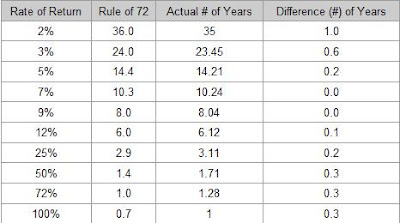## Tuesday, July 27, 2010

### What is the 'Rule of 72'?The 'Rule of 72' is a simplified way to determine how long an investment will take to double, given a fixed annual rate of interest. By dividing 72 by the annual rate of return, investors can get a rough estimate of how many years it will take for the initial investment to duplicate itself.

Step 1: How long does it take my money to double?

Divide the number 72 by the percentage rate you are paying on your debt, or earning on your investment. Here are two examples...

You borrowed \$1,000 from your friend, who is charging you 6% interest. 72 divided by 6 is 12. That makes 12 the number of years it would take for your debt to your friend to double to \$2,000 if you did not make any payments.

You have a savings account with \$500 deposited in it. It earns 4% interest from the bank. 72 divided by 4 is 18. It will take 18 years for your \$500 to double to \$1,000 if you don't make any deposits.

Remember: 72 divided by the Interest Percentage is the number of years it takes to double.

Step 2: How many times will my money double?

This step teaches you how important it is for your money to double as many times as possible, and for your debts to double as few times as possible.

Determine how many years you will keep your investment before cashing it in. Divide that by the number of years it will take to double each time, the number you figured out in step one.

Now look at what happens to your money each time it doubles...

\$1 ... \$2 ... \$4 ... \$8 ... \$16 ... \$32 ... \$64 ... \$128 ...

You can see that it makes a big difference how many times your money doubles. If you can make it double only a few more times by making just slightly better investments, you can end up with many times more money at retirement, or whenever you cash in your investment.

Think about how fast your debts can double with high interest rates, such as those charged on most credit card accounts.

When dealing with low rates of return, the Rule of 72 is fairly accurate. This chart compares the numbers given by the rule of 72 and the actual number of years it takes an investment to double.For example, the rule of 72 states that \$1 invested at 10% would take 7.2 years ((72/10) = 7.2) to turn into \$2. In reality, a 10% investment will take 7.3 years to double ((1.10^7.3 = 2).

Notice that, although it gives a quick rough estimate, the rule of 72 gets less precise as rates of return become higher. Therefore, when dealing with higher rates, it's best to calculate the precise number of years algebraically by means of the future value formula.

Source:
Investopedia, ruleof72.net